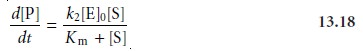Home | | Modern Analytical Chemistry | Methods Based on Chemical Kinetics: Quantitative Applications

# Methods Based on Chemical Kinetics: Quantitative Applications

Chemical kinetic methods of analysis continue to find use for the analysis of a vari- ety of analytes, most notably in clinical laboratories, where automated methods aid in handling a large volume of samples.

Quantitative Applications

Chemical kinetic methods of analysis continue to find use for the analysis of a vari- ety of analytes, most notably in clinical laboratories, where automated methods aid in handling a large volume of samples. In this section several general quantitative applications are considered.

## Enzyme-Catalyzed Reactions

Enzymes are highly specific catalysts for biochemi- cal reactions, with each enzyme showing a selectivity for a single reactant, or sub- strate. For example, acetylcholinesterase is an enzyme that catalyzes the decomposi- tion of the neurotransmitter acetylcholine to choline and acetic acid. Many enzyme–substrate reactions follow a simple mechanism consisting of the initial for- mation of an enzyme–substrate complex, ES, which subsequently decomposes to form product, releasing the enzyme to react again.If measurements are made early in the reaction, the product’s concentration is neg- ligible, and the step described by the rate constant k–2 can be ignored. Under these conditions the rate of the reaction isTo be analytically useful equation 13.16 needs to be written in terms of the concentrations of enzyme and substrate. This is accomplished by applying the “steady-state” approximation, in which we assume that the concentration of ES is essentially constant. After an initial period in which the enzyme–substrate complex first forms, the rate of formation of ESare equal. Combining these equations gives

k1([E]0 – [ES])[S] = k–1[ES] + k2[ES]

which is solved for the concentration of the enzyme–substrate complexwhere Km is called the Michaelis constant. Substituting equation 13.17 into equa- tion 13.16 leads to the final rate equationA plot of equation 13.18, shown in Figure 13.10, is instructive for defining con- ditions under which the rate of an enzymatic reaction can be used for the quantita- tive analysis of enzymes and substrates. For high substrate concentrations, where [S] >> Km, equation 13.18 simplifies towhere Vmax is the maximum rate for the catalyzed reaction. Under these conditions the rate of the reaction is pseudo-zero-order in substrate, and the maximum rate can be used to calculate the enzyme’s concentration. Typically, this determination is made by a variable-time method. At lower substrate concentrations, where
[S] << Km, equation 13.18 becomesThe reaction is now first-order in substrate, and the rate of the reaction can be used to determine the substrate’s concentration by a fixed-time method.

Chemical kinetic methods have been applied to the quantitative analysis of a number of enzymes and substrates.13 One example, is the determination of glucose based on its oxidation by the enzyme glucose oxidase.Conditions are controlled, such that equation 13.20 is valid. The reaction is moni- tored by following the rate of change in the concentration of dissolved O2 using an appropriate voltammetric technique.

## Nonenzyme-Catalyzed Reactions

The variable-time method has also been used to determine the concentration of nonenzymatic catalysts. Because a trace amount of catalyst can substantially enhance a reaction’s rate, a kinetic determination of a cat- alyst’s concentration is capable of providing an excellent detection limit. One of the most commonly used reactions is the reduction of H2O2 by reducing agents, such as thiosulfate, iodide, and hydroquinone. These reactions are catalyzed by trace levels of selected metal ions. For example the reduction of H2O2 by I

2I+ H2O2 + 2H3O+ < = = = = > 2H2O+ I2

is catalyzed by Mo(VI), W(VI), and Zr(IV). A variable-time analysis is conducted by adding a small, fixed amount of ascorbic acid to each solution. As I2 is produced, it rapidly oxidizes the ascorbic acid and is, itself, reduced back to I. Once all the ascorbic acid is consumed, the presence of excess I2 provides a visual end point.

## Noncatalytic Reactions

Chemical kinetic methods are not as common for the quantitative analysis of analytes in noncatalytic reactions. Because they lack the en- hancement of reaction rate obtained when using a catalyst, noncatalytic methods generally are not used for the determination of analytes at low concentrations.4 Noncatalytic methods for analyzing inorganic analytes are usually based on a com- plexation reaction. One example was outlined in Example 13.4, in which the con- centration of aluminum in serum was determined by the initial rate of formation of its complex with 2-hydroxy-1-naphthaldehyde p-methoxybenzoyl-hydrazone.10 The greatest number of noncatalytic methods, however, are for the quantitative analysis of organic analytes. For example, the insecticide methyl parathion has been determined by measuring its rate of hydrolysis in alkaline solutions.

Study Material, Lecturing Notes, Assignment, Reference, Wiki description explanation, brief detail
Modern Analytical Chemistry: Kinetic Methods of Analysis : Methods Based on Chemical Kinetics: Quantitative Applications |